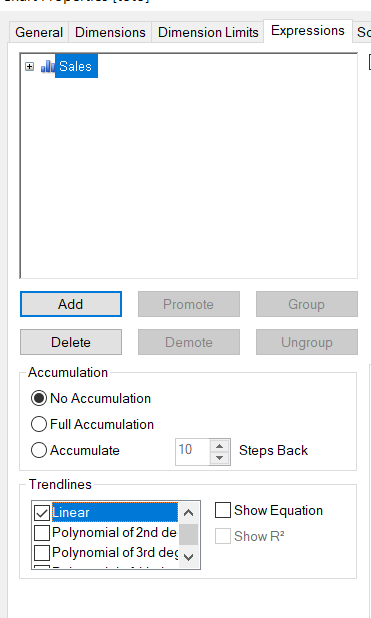New to QlikView

Discussion board where members can get started with QlikView.

Announcements
QlikWorld 2020: Join us May 11 - 14, 2020 in Phoenix, AZ. Register early and save \$400. Learn More
Highlighted
New Contributor

How to add a linear function in graph

Hi,

I would like to add the linear regression y=x to my existing graph:Also, would you know a way to calculate the area under the curve ?

Many thanks,

Lea

6 Replies
Honored Contributor II

Re: How to add a linear function in graph

i do not think so , directly not possible..,so lets wait for some one reply

if u r asking any statistical tools (like R),so u can map and do whatever u need.

Valued Contributor II

Re: How to add a linear function in graph

Hi, you can add linear function with Properties/Expressions/TrendlinesNew Contributor

Re: How to add a linear function in graph

Hi,

I need to add the linear function x=y which is not connected to my expression.

I would like to create another linear trend in the same graph which is equal to:

X, Y,

0, 0%

1, 10%

2, 20%,

3, 30%,

4, 40%

5, 50%

6, 60%,

7, 70%,

8, 80%

9, 90%

10, 100%

Valued Contributor II

Re: How to add a linear function in graph

Hi,

between two deciles :

the area (approx) is

(y + below(y))/2 * 1 ( 1 = diff between two decile)

you sum this expression and you have the area under the curve

if you want to do this for your linear regression : look at linest_   functions

you can use too 1/100 fractile to be more precise

regards

Valued Contributor II

Re: How to add a linear function in graph

Valued Contributor II

Re: How to add a linear function in graph

here is explanation of area calculation,

made it several times for lorentz/pareto curve or roc curvefor y=x

maybe somethink like (rowno -1) * 10  as far as X is 0 to 10 ?

tell me is that suit you